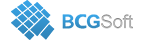BCGControlBar Pro for MFC
 CBCGPChartBaseFormula* CBCGPChartSeries::GetFormula ( ) const

Returns a formula used to generate series data points.

Call this method to retrieve a pointer to a formula used to generate series data point. If you use a class derived from CBCGPChartAdvancedFormula, then the formula object was set automatically by CBCGPChartAdvancedFormula::Create. For other formula types (CBCGPChartTransitionFormula, CBCGPChartTrendFormula) you should call CBCGPChartSeries::SetFormula explicitly.